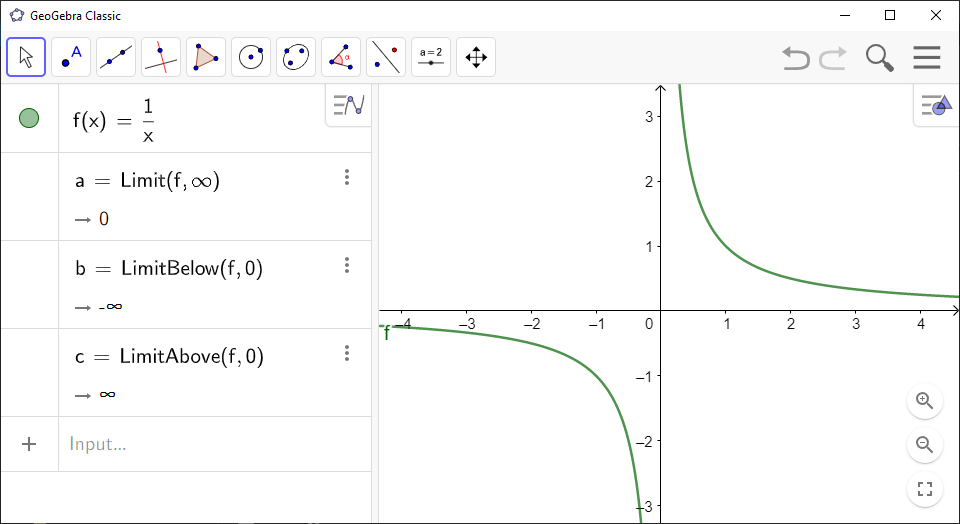# How to Calculate Limits of Functions Using GeoGebra

You can use `GeoGebra` to compute limit of function as the variable tends to a certain value.

`GeoGebra` Instruction 1

Open `Algebra View` under`View` in`Menu`.

• To find the regular two-sided limit of `<Function>` as the variable approaches `<Value>`, use the command

Limit(<Function>, <Value>)

• To find the left-handed limit, use the command

LimitBelow(<Function>, <Value>)

• To find the right-handed limit, use the command

LimitAbove(<Function>, <Value>)